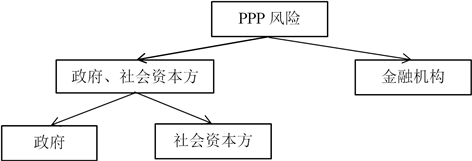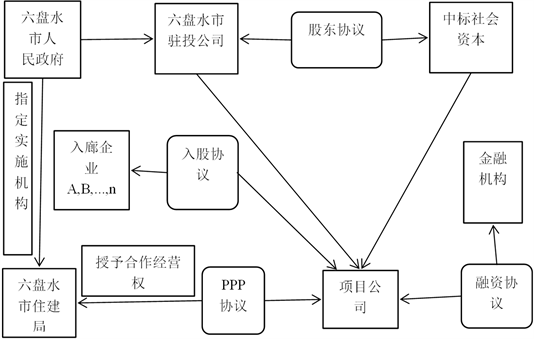﻿ 动态博弈视角下地下综合管廊PPP项目风险分担

# 动态博弈视角下地下综合管廊PPP项目风险分担Risk Sharing of Underground Integrated Pipe Gallery PPP Project from the Perspective of Dynamic Game

Abstract: The comprehensive pipe corridor PPP project has many complicated risks, and it involves a large number of participants. How to design a complete set of system scientific and suitable for China’s current development of the national risk sharing mechanism is crucial to ensure the successful construction of the integrated pipe PPP project. In view of this, this paper proposes to construct a dynamic bargaining game model of government departments, social capital parties and financial institutions under incomplete information. First, the first phase of negotiations between the “alliance” composed of government and social capital parties and financial institutions is carried out. Then, the game is divided into the risk distribution between the government and the social capital, so as to calculate the risk sharing ratio of the participants in the PPP project. Finally, the model is applied to the PPP project of the underground comprehensive pipe gallery in Liupanshui City, Guizhou Province, to verify the rationality of the risk sharing model.

1. 引言

2. 博弈过程Figure 1. Three-party game model for risk sharing of integrated pipe corridor PPP project

3. 不完全信息下三方风险分担的第一阶段模型构建及分析

3.1. 模型假设

3.2. 模型参数的设定

1) 谈判损耗系数 $\mu$ 。在“同盟”与金融机构讨价还价的谈判过程中，谈判损耗系数( $\mu >1$ )是其中最重要的一个参数。在双方博弈过程中必然会耗费一定的谈判成本，主要包括时间成本、机会成本等，这些成本会随着谈判次数的增加而增多。在实际中由于双方地位等客观因素的影响，使得“同盟”的谈判损耗系数 ${\mu }_{a}$ 小于金融机构的谈判损耗系 ${\mu }_{b}$

2) 风险转移因子 $\beta$ 。因为“同盟”是由政府和社会资本方构成的，势必处于强势地位。在这里当“同盟”具有高威慑力时，将以概率p的可能性威慑对方接纳自己的部分风险 ${\beta }_{i}^{H}$ ，而当它具有低威慑力时则以概率 $1-p$ 的可能性威慑对方接纳自己的部分风险 ${\beta }_{i}^{L}$ ，其中 ${\beta }_{i}^{H}>{\beta }_{i}^{L}$ ，且风险转移因子小于各自承担的风险比例，即 $\text{0}\le {\beta }_{i}^{m}\le {k}_{i}<1,m=H,L;i=1,2,3$

3.3. 模型的构建

${{A}^{\prime }}_{1}=p\left({k}_{1}-{\beta }_{1}^{H}\right),\text{ }{{B}^{\prime }}_{1}=p\left(1-{k}_{1}+{\beta }_{1}^{H}\right)$ (1)

${{A}^{″}}_{1}=\left(1-p\right)\left({k}_{1}-{\beta }_{1}^{L}\right),\text{ }{{B}^{″}}_{1}=\left(1-p\right)\left(1-{k}_{1}+{\beta }_{1}^{L}\right)$ (2)

${A}_{1}={{A}^{\prime }}_{1}+{{A}^{″}}_{1}=p\left({k}_{1}-{\beta }_{1}^{H}\right)+\left(1-p\right)\left({k}_{1}-{\beta }_{1}^{L}\right)$ (3)

${B}_{1}={{B}^{\prime }}_{1}+{B}^{″}=p\left(1-{k}_{1}+{\beta }_{1}^{H}\right)+\left(1-p\right)\left(1-{k}_{1}+{\beta }_{1}^{L}\right)$ (4)

${{A}^{\prime }}_{\text{2}}=p{\mu }_{a}\left({k}_{\text{2}}-{\beta }_{\text{2}}^{H}\right),\text{ }{{B}^{\prime }}_{\text{2}}=p{\mu }_{b}\left(1-{k}_{\text{2}}+{\beta }_{\text{2}}^{H}\right)$ (5)

${{A}^{″}}_{\text{2}}=\left(1-p\right){\mu }_{a}\left({k}_{\text{2}}-{\beta }_{\text{2}}^{L}\right),\text{ }{{B}^{″}}_{\text{2}}=\left(1-p\right){\mu }_{b}\left(1-{k}_{\text{2}}+{\beta }_{\text{2}}^{L}\right)$ (6)

${A}_{2}={A}^{\prime }+{{A}^{″}}_{\text{2}}=p{\mu }_{a}\left({k}_{\text{2}}-{\beta }_{\text{2}}^{H}\right)+\left(1-p\right){\mu }_{a}\left({k}_{\text{2}}-{\beta }_{\text{2}}^{L}\right)$ (7)

${B}_{2}={{B}^{\prime }}_{\text{2}}+{{B}^{″}}_{\text{2}}=p{\mu }_{b}\left(1-{k}_{\text{2}}+{\beta }_{\text{2}}^{H}\right)+\left(1-p\right){\mu }_{b}\left(1-{k}_{\text{2}}+{\beta }_{\text{2}}^{L}\right)$ (8)

${{A}^{\prime }}_{\text{3}}=p{\mu }_{a}^{\text{2}}\left({k}_{\text{3}}-{\beta }_{\text{3}}^{H}\right),\text{ }{{B}^{\prime }}_{\text{3}}=p{\mu }_{b}^{2}\left(1-{k}_{\text{3}}+{\beta }_{\text{3}}^{H}\right)$ (9)

${{A}^{″}}_{\text{3}}=\left(1-p\right){\mu }_{a}^{\text{2}}\left({k}_{\text{3}}-{\beta }_{\text{3}}^{L}\right),\text{ }{{B}^{″}}_{\text{3}}=\left(1-p\right){\mu }_{b}^{\text{2}}\left(1-{k}_{\text{3}}+{\beta }_{\text{3}}^{L}\right)$ (10)

${A}_{\text{3}}={{A}^{\prime }}_{\text{3}}+{{A}^{″}}_{\text{3}}=p{\mu }_{a}^{\text{2}}\left({k}_{\text{3}}-{\beta }_{\text{3}}^{H}\right)+\left(1-p\right){\mu }_{a}^{\text{2}}\left({k}_{\text{3}}-{\beta }_{\text{3}}^{L}\right)$ (11)

${B}_{\text{3}}={{B}^{\prime }}_{\text{3}}+{{B}^{″}}_{\text{3}}=p{\mu }_{b}^{2}\left(1-{k}_{\text{3}}+{\beta }_{\text{3}}^{H}\right)+\left(1-p\right){\mu }_{b}^{\text{2}}\left(1-{k}_{\text{3}}+{\beta }_{\text{3}}^{L}\right)$ (12)

3.4. 模型求解

${A}_{2}={A}_{3}$ (13)

$p{\mu }_{a}\left({k}_{2}-{\beta }_{2}^{H}\right)+\left(1-p\right){\mu }_{a}\left({k}_{2}-{\beta }_{2}^{L}\right)=p{\mu }_{a}^{2}\left({k}_{3}-{\beta }_{2}^{H}\right)+\left(1-p\right){\mu }_{a}^{2}\left({k}_{3}-{\beta }_{3}^{L}\right)$ (14)

${k}_{2}=p{\beta }_{2}^{H}+\left(1-p\right){\beta }_{2}^{L}+p{\mu }_{a}\left({k}_{3}-{\beta }_{3}^{H}\right)+\left(1-p\right){\mu }_{a}\left({k}_{3}-{\beta }_{3}^{L}\right)$ (15)

${B}_{2}={\mu }_{b}-p{\mu }_{a}{\mu }_{b}\left({k}_{3}-{\beta }_{3}^{H}\right)-\left(1-p\right){\mu }_{a}{\mu }_{b}\left({k}_{3}-{\beta }_{3}^{L}\right)$ (16)

${B}_{2}-{B}_{3}={\mu }_{b}\left(1-{\mu }_{b}\right)+{\mu }_{b}{k}_{3}\left({\mu }_{b}-{\mu }_{a}\right)+p{\mu }_{b}\left({\mu }_{a}-{\mu }_{b}\right){\beta }_{3}^{H}+\left(1-p\right){\mu }_{b}\left({\mu }_{a}-{\mu }_{b}\right){\beta }_{3}^{L}$ (17)

${B}_{1}={B}_{2}$ (18)

$p\left(1-{k}_{1}+{\beta }_{1}^{H}\right)+\left(1-p\right)\left(1-{k}_{1}+{\beta }_{1}^{L}\right)={\mu }_{b}-p{\mu }_{a}{\mu }_{b}\left({k}_{3}-{\beta }_{2}^{H}\right)-\left(1-p\right){\mu }_{a}{\mu }_{b}\left({k}_{3}-{\beta }_{2}^{L}\right)$ (19)

${k}_{1}=1-{\mu }_{b}+p{\beta }_{1}^{H}+\left(1-p\right){\beta }_{1}^{L}+p{\mu }_{a}{\mu }_{b}\left({k}_{3}-{\beta }_{3}^{H}\right)+\left(1-p\right){\mu }_{a}{\mu }_{b}\left({k}_{3}-{\beta }_{3}^{L}\right)$ (20)

${k}_{1}={k}_{3}$ ，将其带入式(20)可得：

${k}^{\ast }=\frac{1-{\mu }_{b}}{1-{\mu }_{a}{\mu }_{b}}+\frac{p\left({\beta }_{1}^{H}-{\mu }_{a}{\mu }_{b}{\beta }_{3}^{H}\right)}{1-{\mu }_{a}{\mu }_{b}}+\frac{\left(1-p\right)\left({\beta }_{1}^{L}-{\mu }_{a}{\mu }_{b}{\beta }_{3}^{L}\right)}{1-{\mu }_{a}{\mu }_{b}}$ (21)

$\text{1}-{k}^{\ast }=\frac{{\mu }_{b}\left(1-{\mu }_{a}\right)}{1-{\mu }_{a}{\mu }_{b}}-\frac{p\left({\beta }_{1}^{H}-{\mu }_{a}{\mu }_{b}{\beta }_{3}^{H}\right)}{1-{\mu }_{a}{\mu }_{b}}-\frac{\left(1-p\right)\left({\beta }_{1}^{L}-{\mu }_{a}{\mu }_{b}{\beta }_{3}^{L}\right)}{1-{\mu }_{a}{\mu }_{b}}$ (22)

$\left\{\begin{array}{l}\frac{{\beta }_{\text{1}}^{H}-{\mu }_{a}{\mu }_{b}{\beta }_{3}^{H}}{1-{\mu }_{a}{\mu }_{b}}={\beta }^{H}\\ \frac{{\beta }_{1}^{L}-{\mu }_{a}{\mu }_{b}{\beta }_{3}^{L}}{1-{\mu }_{a}{\mu }_{b}}={\beta }^{L}\end{array}$ (23)

“同盟”： ${k}^{\ast }=\frac{1-{\mu }_{b}}{1-{\mu }_{a}{\mu }_{b}}+p{\beta }^{H}+\left(1-p\right){\beta }^{L}$

4. 不完全信息下三方风险分担的第二阶段模型构建及分析

4.1. 模型假设

4.2. 模型参数的设定

1) 谈判损耗系数 $\phi$ 。在实际城市地下综合管廊PPP项目谈判过程中，政府相对于社会资本方占据优势地位，所以每进行一次博弈政府需要承担的谈判成本都会小于社会资本方的谈判成本，故政府的谈判损耗系数 ${\phi }_{g}$ 低于社会资本方的谈判损耗系数 ${\phi }_{e}$ ，即 ${\phi }_{e}>{\phi }_{g}>1,{\mu }_{a}=\left({\phi }_{e}+{\phi }_{g}\right)/2$

2) 风险转移因子 $\theta$ 。政府势必处于强势地位，它具有高威慑力和低威慑力两种类型。当政府具有高威慑力时，将以概率q的可能性威慑对方接受自己的部分风险 ${\theta }_{i}^{H}$ ，而当它具有低威慑力时则以概率 $1-q$ 的可能性威慑对方接受自己的部分风险 ${\theta }_{i}^{L}$ ，其中 ${\theta }_{i}^{H}>{\theta }_{i}^{L}$ ，且风险转移因子小于各自承担的风险比例，即 $\text{0}\le {\theta }_{i}^{m}\le {\eta }_{i}<1,m=H,L;i=1,2,3$

4.3. 模型的构建

${{G}^{\prime }}_{1}=q\left({\eta }_{1}-{\theta }_{1}^{H}\right),\text{ }{{E}^{\prime }}_{1}=q\left(k-{\eta }_{1}+{\theta }_{1}^{H}\right)$ (24)

${{G}^{″}}_{1}=\left(1-q\right)\left({\eta }_{1}-{\theta }_{1}^{L}\right),\text{ }{{E}^{″}}_{1}=\left(1-q\right)\left(k-{\eta }_{1}+{\theta }_{1}^{L}\right)$ (25)

${G}_{1}={{G}^{\prime }}_{1}+{{G}^{″}}_{1}=q\left({\eta }_{1}-{\theta }_{1}^{H}\right)+\left(1-q\right)\left({\eta }_{1}-{\theta }_{1}^{L}\right)$ (26)

${E}_{1}={{E}^{\prime }}_{1}+{{E}^{″}}_{1}=q\left(k-{\eta }_{1}+{\theta }_{1}^{H}\right)+\left(1-q\right)\left(k-{\eta }_{1}+{\theta }_{1}^{L}\right)$ (27)

${{G}^{\prime }}_{\text{2}}=q{\phi }_{g}\left({\eta }_{2}-{\theta }_{2}^{H}\right),\text{ }{{E}^{\prime }}_{\text{2}}=q{\phi }_{e}\left(k-{\eta }_{2}+{\theta }_{2}^{H}\right)$ (28)

${{G}^{″}}_{\text{2}}=\left(1-q\right){\phi }_{g}\left({\eta }_{2}-{\theta }_{2}^{L}\right),\text{ }{{E}^{″}}_{\text{2}}=\left(1-q\right){\phi }_{e}\left(k-{\eta }_{2}+{\theta }_{2}^{L}\right)$ (29)

${G}_{\text{2}}={{G}^{\prime }}_{\text{2}}+{{G}^{″}}_{\text{2}}=q{\phi }_{g}\left({\eta }_{2}-{\theta }_{2}^{H}\right)+\left(1-q\right){\phi }_{g}\left({\eta }_{2}-{\theta }_{2}^{L}\right)$ (30)

${E}_{\text{2}}={{E}^{\prime }}_{\text{2}}+{{E}^{″}}_{\text{2}}=q{\phi }_{e}\left(k-{\eta }_{2}+{\theta }_{2}^{H}\right)+\left(1-q\right){\phi }_{e}\left(k-{\eta }_{2}+{\theta }_{2}^{L}\right)$ (31)

${{G}^{\prime }}_{\text{3}}=q{\phi }_{g}^{2}\left({\eta }_{\text{3}}-{\theta }_{\text{3}}^{H}\right),\text{ }{{E}^{\prime }}_{\text{3}}=q{\phi }_{e}^{2}\left(k-{\eta }_{\text{3}}+{\theta }_{\text{3}}^{H}\right)$ (32)

${{G}^{″}}_{\text{3}}=\left(1-q\right){\phi }_{g}^{2}\left({\eta }_{3}-{\theta }_{3}^{L}\right),\text{ }{{E}^{″}}_{\text{3}}=\left(1-q\right){\phi }_{e}^{2}\left(k-{\eta }_{\text{3}}+{\theta }_{\text{3}}^{L}\right)$ (33)

${G}_{\text{3}}={{G}^{\prime }}_{\text{3}}+{{G}^{″}}_{\text{3}}=q{\phi }_{g}^{2}\left({\eta }_{\text{3}}-{\theta }_{\text{3}}^{H}\right)+\left(1-q\right){\phi }_{g}^{2}\left({\eta }_{3}-{\theta }_{3}^{L}\right)$ (34)

${E}_{\text{3}}={{E}^{\prime }}_{\text{3}}+{{E}^{″}}_{\text{3}}=q{\phi }_{e}^{2}\left(k-{\eta }_{\text{3}}+{\theta }_{\text{3}}^{H}\right)+\left(1-q\right){\phi }_{e}^{2}\left(k-{\eta }_{\text{3}}+{\theta }_{\text{3}}^{L}\right)$ (35)

4.4. 模型求解

${G}_{2}={G}_{3}$ (36)

$q{\phi }_{g}\left({\eta }_{2}-{\theta }_{2}^{H}\right)+\left(1-q\right){\phi }_{g}\left({\eta }_{2}-{\theta }_{2}^{L}\right)=q{\phi }_{g}^{2}\left({\eta }_{3}-{\theta }_{3}^{H}\right)+\left(1-q\right){\phi }_{g}^{2}\left({\eta }_{3}-{\theta }_{3}^{L}\right)$ (37)

${\eta }_{2}=q{\theta }_{2}^{H}+\left(1-q\right){\theta }_{2}^{L}+q{\phi }_{g}\left({\eta }_{3}-{\theta }_{3}^{H}\right)+\left(1-q\right){\phi }_{g}\left({\eta }_{3}-{\theta }_{3}^{L}\right)$ (38)

${E}_{2}=k{\phi }_{e}-{\phi }_{e}{\phi }_{g}{\eta }_{3}+q{\phi }_{e}{\phi }_{g}{\theta }_{3}^{H}+\left(1-q\right){\phi }_{e}{\phi }_{g}{\theta }_{3}^{L}$ (39)

${E}_{2}-{E}_{3}=k{\phi }_{e}\left(1-{\phi }_{e}\right)+{\phi }_{e}{\eta }_{3}\left({\phi }_{e}-{\phi }_{g}\right)+q{\phi }_{e}\left({\phi }_{g}-{\phi }_{e}\right){\theta }_{3}^{H}+\left(1-q\right){\phi }_{e}\left({\phi }_{g}-{\phi }_{e}\right){\theta }_{3}^{L}$ (40)

${E}_{1}={E}_{2}$ (41)

$q\left(k-{\eta }_{1}+{\theta }_{1}^{H}\right)+\left(1-q\right)\left(k-{\eta }_{1}+{\theta }_{1}^{L}\right)=k{\phi }_{e}-{\phi }_{e}{\phi }_{g}{\eta }_{3}+q{\phi }_{e}{\phi }_{g}{\theta }_{3}^{H}+\left(1-q\right){\phi }_{e}{\phi }_{g}{\theta }_{3}^{L}$ (42)

${\eta }_{\text{1}}=k-k{\phi }_{e}+q{\theta }_{1}^{H}+\left(1-q\right){\theta }_{1}^{L}+{\phi }_{e}{\phi }_{g}{\eta }_{3}-q{\phi }_{e}{\phi }_{g}{\theta }_{3}^{H}-\left(1-q\right){\phi }_{e}{\phi }_{g}{\theta }_{3}^{L}$ (43)

${\eta }_{1}={\eta }_{3}$ ，将其带入式(43)可得：

${\eta }^{\ast }=\frac{\left(1-{\phi }_{e}\right)k}{1-{\phi }_{e}{\phi }_{g}}+\frac{q\left({\theta }_{1}^{H}-{\phi }_{e}{\phi }_{g}{\theta }_{3}^{H}\right)}{1-{\phi }_{e}{\phi }_{g}}+\frac{\left(1-q\right)\left({\theta }_{1}^{L}-{\phi }_{e}{\phi }_{g}{\theta }_{3}^{L}\right)}{1-{\phi }_{e}{\phi }_{g}}$ (44)

$k-{\eta }^{\ast }=\frac{{\phi }_{e}\left(1-{\phi }_{g}\right)k}{1-{\phi }_{e}{\phi }_{g}}-\frac{q\left({\theta }_{1}^{H}-{\phi }_{e}{\phi }_{g}{\theta }_{3}^{H}\right)}{1-{\phi }_{e}{\phi }_{g}}-\frac{\left(1-q\right)\left({\theta }_{1}^{L}-{\phi }_{e}{\phi }_{g}{\theta }_{3}^{L}\right)}{1-{\phi }_{e}{\phi }_{g}}$ (45)

$\left\{\begin{array}{l}\frac{{\theta }_{1}^{H}-{\phi }_{e}{\phi }_{g}{\theta }_{3}^{H}}{1-{\phi }_{e}{\phi }_{g}}={\theta }^{H}\\ \frac{{\theta }_{1}^{L}-{\phi }_{e}{\phi }_{g}{\theta }_{3}^{L}}{1-{\phi }_{e}{\phi }_{g}}={\theta }^{L}\end{array}$ (46)

${\mu }_{a}=\left({\phi }_{e}+{\phi }_{g}\right)/2$ 和k带入可得政府部门、社会资本方和金融机构的风险分担比例依次为：

${\eta }^{\ast }=\left(1-{\phi }_{e}\right)\left\{\frac{2\left(1-{\mu }_{b}\right)}{\left(1-{\phi }_{e}{\phi }_{g}\right)\left[2-\left({\phi }_{e}+{\phi }_{g}\right){\mu }_{b}\right]}+\frac{p{\beta }^{H}+\left(1-p\right){\beta }^{L}}{1-{\phi }_{e}{\phi }_{g}}\right\}+q{\theta }^{H}+\left(1-q\right){\theta }^{L}$ (47)

$k-{\eta }^{\ast }={\phi }_{e}\left(1-{\phi }_{g}\right)\left\{\frac{2\left(1-{\mu }_{b}\right)}{\left(1-{\phi }_{e}{\phi }_{g}\right)\left[2-\left({\phi }_{e}+{\phi }_{g}\right){\mu }_{b}\right]}+\frac{p{\beta }^{H}+\left(1-p\right){\beta }^{L}}{1-{\phi }_{e}{\phi }_{g}}\right\}-q{\theta }^{H}-\left(1-q\right){\theta }^{L}$ (48)

$1-{k}^{\ast }=\frac{\left(2-{\phi }_{e}-{\phi }_{g}\right){\mu }_{b}}{2-\left({\phi }_{e}+{\phi }_{g}\right){\mu }_{b}}-p{\beta }^{H}-\left(1-p\right){\beta }^{L}$ (49)

5. 实例分析

5.1. 项目概况Figure 2. Liupanshui City pipe gallery PPP project operation process

5.2. 风险分担思路及风险因素识别Table 1. Risk sharing of PPP project in Liupanshui City pipeline

5.3. 风险分担模型参数的确定和分担比例的计算Table 2. Risk-related parameters of the PPP project of Liupanshui City pipelineTable 3. Tripartite risk sharing ratio results

6. 结论

 Medda, F. (2007) A Game Theory Approach for the Allocation of Risks in Transport Public Private Partnerships. Science Direct, 25, 213-218.
https://doi.org/10.1016/j.ijproman.2006.06.003

 薛琳婧. PPP模式下综合管廊项目风险分担模型研究[D]: [硕士学位论文]. 西安: 西安建筑科技大学, 2017.

 汪婷. 地下综合管廊PPP项目风险分担研究[D]: [硕士学位论文]. 武汉: 武汉大学, 2018.

 有维宝, 王建波, 张樵民, 等. 基于TOPSIS-UT的城市地下综合管廊PPP项目风险分担[J]. 土木工程与管理学报, 2019, 36(2): 186-194.

Top